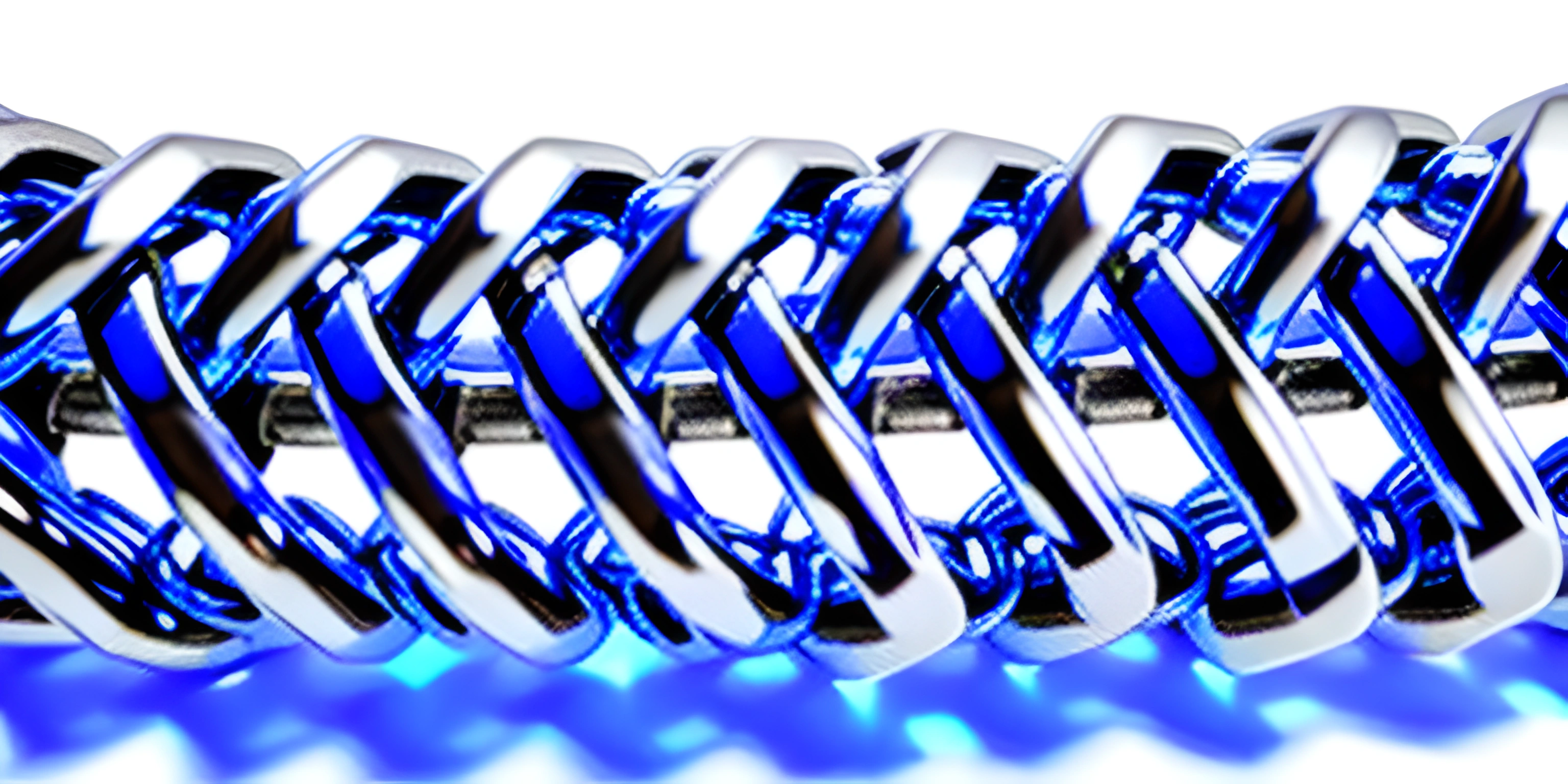Note: this page has been created with the use of AI. Please take caution, and note that the content of this page does not necessarily reflect the opinion of Cratecode.

If you've ever found yourself yearning for a more dynamic and flexible approach to storing data, then it's time to get acquainted with linked lists. Linked lists are an essential data structure in programming that can be used to store and manipulate collections of elements.

## What is a Linked List?

A linked list is a linear data structure in which elements are stored in nodes, and each node points to the next node in the sequence. The first element is called the head of the list, and the last element is called the tail. The tail node has a reference to `null`, indicating the end of the list.

Let's take a look at a simple example. Say we have a list of numbers: `[3, 7, 12]`. In a linked list, it would be represented as follows:

``HEAD:  3  ->  7  ->  12  ->  NULL``

Linked lists have some unique advantages over other data structures like arrays:

1. Dynamic size: Linked lists can grow or shrink in size as needed, making them perfect for situations where the number of elements is unknown or constantly changing.
2. Flexible memory allocation: Unlike arrays, linked list nodes don't need to be stored in contiguous memory locations, allowing for more efficient use of memory.
3. Efficient insertions and deletions: Adding or removing elements from a linked list can be done in constant time, as long as we have a reference to the node to be modified.

However, linked lists also come with their share of drawbacks:

1. Increased memory overhead: Each node in a linked list requires an extra reference to the next node, increasing the memory usage.
2. Slow random access: Accessing elements in a linked list takes linear time, as we have to traverse the list from the head until we reach the desired node.
3. Difficulty with reverse traversal: Unlike arrays or doubly linked lists, singly linked lists cannot be traversed in reverse without significant modifications.

Now that we've covered the basics, let's dive into some common operations on linked lists:

To add an element to a linked list, we can either insert it at the beginning (head) or the end (tail) of the list. Let's look at an example in Python:

``````class Node:
def __init__(self, data):
self.data = data
self.next = None

def __init__(self):

def insert_at_beginning(self, data):
new_node = Node(data)

def insert_at_end(self, data):
new_node = Node(data)

return

while current.next:
current = current.next
current.next = new_node``````

### Removing an Element

To remove an element from a linked list, we need to find the node before the one we want to remove and update its `next` reference. Here's an example in Python:

``````class LinkedList:
# ... Previous code ...

def remove(self, data):
return

return

while current.next and current.next.data != data:
current = current.next

if current.next:
current.next = current.next.next``````

### Searching for an Element

To search for a specific element in a linked list, we start at the head and traverse the nodes until we find the desired data or reach the end of the list. Here's how it's done in Python:

``````class LinkedList:
# ... Previous code ...

def search(self, data):
while current:
if current.data == data:
return True
current = current.next
return False``````

That's a brief introduction to linked lists, their advantages, disadvantages, and some of the operations you'll commonly perform with them. As you explore more advanced programming concepts, you'll no doubt encounter linked lists in various forms, making them a valuable addition to your toolkit.

## FAQ

### What is a linked list and why should I use one?

A linked list is a linear data structure where each element, called a node, points to the next element in the sequence. It's different from arrays, as elements are not stored in contiguous memory locations. Linked lists provide flexibility in memory allocation and are particularly useful for situations where the size of the data set is unknown or changes frequently. However, they have a higher overhead and slower random access times compared to arrays.

### How do I create a basic linked list node in Python?

To create a simple linked list node in Python, you can define a class with a constructor that initializes the node's data and a pointer to the next node. Here's an example:

``````class Node:
def __init__(self, data):
self.data = data
self.next = None``````

### How do I insert a new node at the beginning of a linked list?

To insert a new node at the beginning of a linked list, you can create a new node and set its 'next' attribute to the current head of the list. Then, update the head to be the new node. Here's an example using a `LinkedList` class:

``````class LinkedList:
def __init__(self):
def insert_at_beginning(self, data):
new_node = Node(data)

### How can I traverse a linked list and print its elements?

To traverse a linked list and print its elements, you can create a temporary pointer variable that starts at the head of the list. Then, iterate through the list by updating the pointer to the next node until the end is reached. Here's an example:

``````def print_linked_list(self):